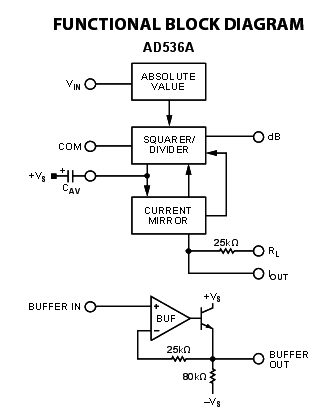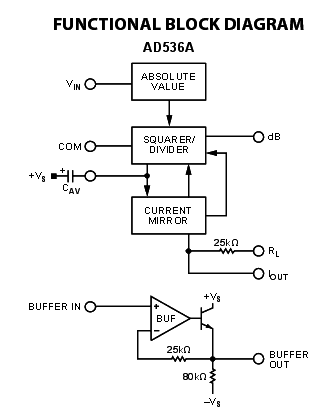Electrical meter and efficiency question

Q1. I know a DMM will anticipate a sineusoidal supply, thus there can be problems with spiky supplies.

Even if you have a true RMS meter, it it's a spikey supply, non-sineusoidal, you still can't calculate the peak of a spiky supply?
Does a DMM measure the peak, then calculate from THAT the rms?
Or does a DMM possibly measure the average and calculate rms from that?

Q2. I've heard that in a TX that maximum efficiency occurs when copper loss is equal to core loss?
Is there a simple reason for this?

Cheers

donpacino
Gold Member
taken off a fluke DMM support sheet

Most DMMs are “average responding,” giving accurate rms readings if the ac voltage signal is a pure sine wave. Average responding meters are not capable of measuring non-sinusoidal signals accurately. Non-sinusoidal signals are accurately measured using DMMs designated “truerms” up to the DMM’s specified crest factor. Crest factor is the ratio of a signal’s peak-to-rms value. It’s 1.414 for a pure sine wave, but is often much higher for a rectifier current pulse, for example. As a result, an average responding meter will often read much lower than the actual rms value

A DMM’s ability to measure ac voltage can be limited by the frequency of the signal. Most DMMs can accurately measure ac voltages with frequencies from 50 Hz to 500 Hz, but a DMM’s ac measurement bandwidth may be hundreds of kilohertz wide. Such a meter may read a higher value because it is “seeing” more of a complex ac signal. DMM accuracy specifications for ac voltage and ac current should state the frequency range along with the range’s accuracy

•davenn
anorlunda
Staff Emeritus
Q1: I don't think you can say that all DMMs use the same methods. But a reaonsable thing for a DMM to do is to put an analog low-pass filter in front before sampling the waveform. They might call it an integrating converter. That would have the effect of converting spikes too fast to measure into averages.

Many modern DVMs use FET circuits which takes them beyond simple linear elements.

https://en.wikipedia.org/wiki/Voltmeter#VTVMs_and_FET-VMs

'Crest factor' that's a nice term, I like it.

Ok, I think you all answered the part about 'it is more likely to calculate the AVERAGE then calculate the RMS from THAT', and may only be ale to capture the peak if it has a special function.
But the other part I'm still not sure about: if you DO have a True RMS meter, can you then calculate the peak from THAT (by multplying by root 2)?

I didn't really understand this converstion to dc diagram:jim hardy
Gold Member
Dearly Missed
But the other part I'm still not sure about: if you DO have a True RMS meter, can you then calculate the peak from THAT (by multplying by root 2)?

RMS to root 2 is for sinewave. see
https://en.wikipedia.org/wiki/Crest_factor

I didn't really understand this converstion to dc diagram:Study text surrounding fig6 page 8 ?
But i think they have a typo, confusing I4 and I2 I2 might have been Ipin4 in an earlier draft ?

•tim9000
Ah ok, yeah I'll have another look when I get a chance.